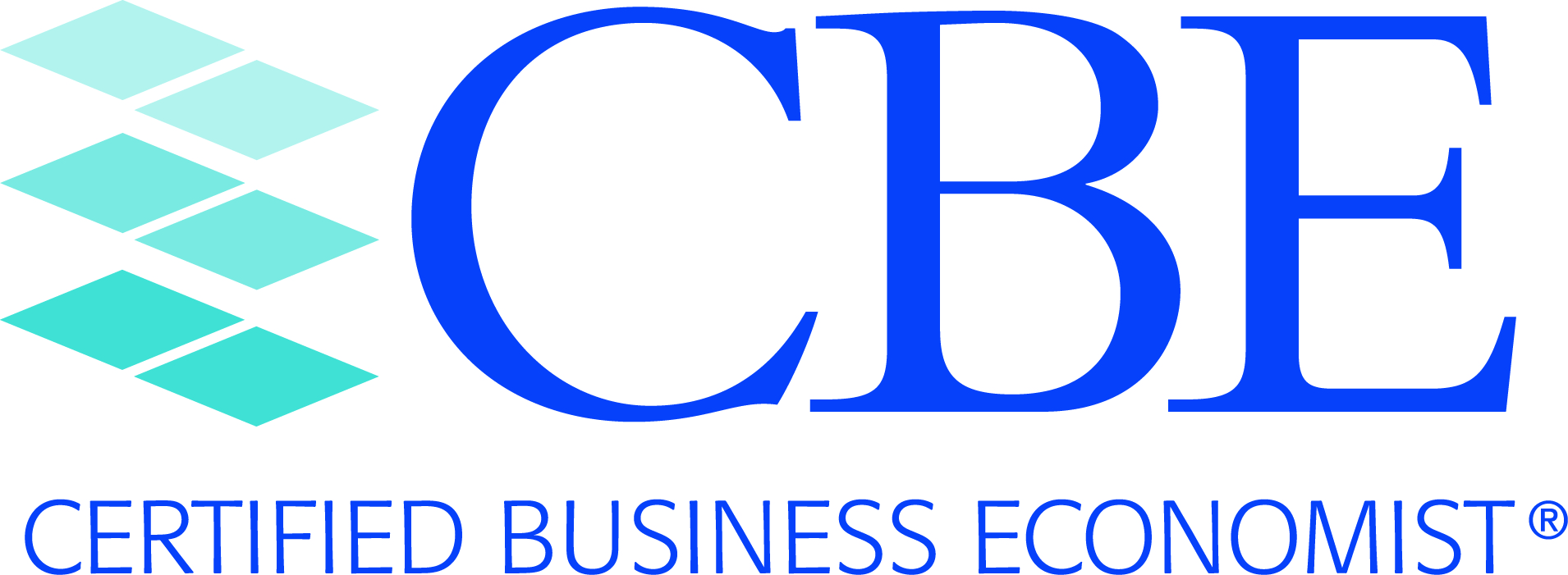Applied Econometrics - Content Outline

1. Getting Started
• The Model Specification Phase
• The Principal of Parsimony
• The Shrinkage Principle
• The Generic Single-Equation Linear Regression Model
• Components of the Model
• Endogenous Variables, Exogenous Variables, Lagged Exogenous Variables, Lagged Endogenous Variables
• The Disturbance (or Error) Term
• Assumptions of Econometric Models
• Data and Data Transformations
• Data Types--Time-Series, Cross-Sectional, Combination
• Getting a Feel for the Data--Plots of Key Variables,Scatter Plots, and Descriptive Statistics
• Massaging the Data
• Expression of Data--Nominal versus Real or Inflation-Adjusted Measures
• Expression of Data--Total or Per Capita Terms
• Expression of Data--Levels, Changes, or Percentage Changes
• Use of Moving Averages or Exponential Smoothing
• Imputations for Missing Data

2. Mathematical and Statistical Considerations
• Key Constructs in Applied Econometric Models
• Estimation of Structural Parameters in Applied Econometrics
• Marginal Effects, Standardized Regression Coefficients. Elasticities, and Partial Correlation Coefficients
• Interval Estimation and the Construction of Confidence Intervals

3. Common Tests of Hypotheses
• Tests of Hypotheses Regarding Structural Parameters of Econometric Models
• Statistical Distributions--Standard Normal, t, Chi-squared, and F
• Level of Significance and p-Values
• Goodness-of-Fit Test
• Tests of Single Coefficients
• Tests of Linear Combinations of Coefficients
• Additional Tests of Hypotheses in Econometric Models
• Test of Normality of the Residuals (the Jarque-Bera Test)
• Ramsey Regression Specification Error Test (the Ramsey RESET Test)
• Box-Cox Test (Test of Functional Form)
• Granger Causality Test (Test of Precedence)
• Hausman Test (Test of Endogeneity/Exogeneity of Explanatory Variables)

4. Use of Indicator or Dummy Variables
• Overview (the Representation of Qualitative Variables in Applied Econometrics)

5. Autocorrelation or Serial Correlation
• Definition, Prevalence, and Consequences of Serial Correlation
• Systematic Pattern in the Residuals
• Positive Versus Negative Autocorrelation
• Formal Tests of Serial Correlation
• Durbin-Watson Test
• Solution to the Serial Correlation Problem

6. Heteroscedasticity
• Definition, Prevalence, and Consequences of Heteroscedasticity
• Indigenous to the Use of Cross-Sectional Data
• Examination of the Residuals--Graphical Depictions
• Formal Tests of Heteroscedasticity
• Solution to the Heteroscedasticity Problem
• Application of Weighted Least Squares

7. Collinearity
• Definition, Prevalence, and Consequences of Collinearity
• Consequences for Structural Parameter Estimates and Forecasting
• Formal Diagnostics of Collinearity

8. Influence Diagnostics: The Detection and Assessment of Data Outliers and Leverage Points
• Definition and Consequences of Influence Points

9. Structural Change and Stability of Structural Coefficients
• Abrupt Structural Change
• Breakpoint(s)
• Use of Sequential Chow Tests
• Formal Tests of Parameter Instability
• Recursive Estimation
• Recursive Residuals
• Solution(s) to Structural Change or Parameter Instability
• Attention to the Time Frame of the Econometric Analysis
• Use of Dummy Variables

10. Distributed Lag Models
• Overview of Distributed Lag Models

11. ARCH and GARCH Models
• Overview of ARCH and GARCH Models
• Mechanics of the Autoregressive Conditional Heteroscedasticity (ARCH) Model
• Stability Considerations
• Mechanics of the Generalized Autoregressive Conditional Heteroscedasticity (GARCH) Model

12. Qualitative Choice Models
• Overview of Qualitative Choice Models
• Binary Choice Models--The Probit Model
• Binary Choice Models--The Logit Model
• Estimation of Probit and Logit Models
• Calculation of Appropriate Marginal Effects
• The Prediction-Success Table

13. Censored Response Models

14. Pooling of Time-Series and Cross-Sectional Data
• Overview and Examples of Pooled Time-Series and Cross-Sectional Data
• To Pool or Not to Pool?
• Cross-Sectionally Heteroscedastic and Time-Wise Autoregressive Models
• Fixed and Random Effects

15. Simultaneous-Equation Models
• Order Conditions for Identification
• Impact, Interim, and Total Multipliers
16. Stability Conditions of Simultaneous-Equation Models

17. Seemingly Unrelated Regression (SUR) Models